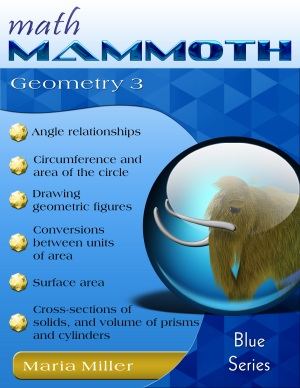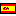^
You are here: HomeBlue Series → Geometry 3

# Math Mammoth Geometry 3128 pages

## Prices & ordering

Printed copy \$16.15

(credit cards, Google Pay, Paypal, PO)Este libro en español

Math Mammoth Geometry 3 can be used after the student has finished Math Mammoth Geometry 1, and is suitable for grades 6-7. This book does not require the students to calculate area or volume, and that is why it is not necessary to study Math Mammoth Geometry 2 (which deals with those topics in depth) before this book.

We start out with basic angle relationships, such as adjacent angles (angles along a line), vertical angles, and corresponding angles (the last only briefly). Classifying triangles according to both angles and sides and the angle sum of a triangle are our next topics, giving students lots of opportunities for drawing exercises. The lesson Angles in Polygons is a sequel to studying angles in a triangle.

The next set of lessons deals with congruent and similar figures. The first lessons in this section are simple enough to be accessible even to 4th-5th grade students. The lesson Similar Figures and Scale Ratio uses the concepts of ratio and proportion, and is meant for 6th-7th graders.

Next, we practice some basic compass-and-ruler constructions. These constructions were practiced and studied a lot by the ancient Greeks. In fact, the mathematics of ancient Greece centered around geometry. Most students will probably enjoy doing the drawing problems in these lessons on blank paper instead of in the worktext. Students also draw figures using a normal ruler and compass in the lesson Drawing Problems. They especially determine whether the given information defines a unique figure (triangle or a parallelogram).

The last section of this book focuses on the Pythagorean Theorem and its applications. First, students need to become familiar with square roots so they can solve the equations that result from applying the Pythagorean Theorem. The first lesson of the section introduces taking a square root as the opposite operation of squaring a number. The lesson includes both a guess and check method and using a calculator to find the square root of a number.

Next, students learn how to solve simple equations that involve taking a square root. This makes them fully ready to study and apply the Pythagorean Theorem. The Pythagorean Theorem is introduced in the lesson with the same name. Students learn to check whether or not a triangle fulfills the Pythagorean Theorem and thus is a right triangle. They apply their knowledge about square roots and solving equations to solve for an unknown side in a right triangle when two of the sides are given.

Next, students solve a variety of real-life and geometric problems that involve the usage of the Pythagorean Theorem. This theorem is extremely important in many practical situations. Students should show their work for these word problems and include the solution process of the equation resulting from the Pythagorean Theorem.

There are literally hundreds of proofs for the Pythagorean Theorem. In this book, we present a proof that is easy and based on geometry (not algebra). Students are asked to supply the steps of reasoning to another, geometric proof of the theorem, and for those interested the lesson also provides an Internet link that has even more proofs of this theorem.

Note: The earlier version of this book (before 8/2015) was shorter and covered less topics.

## Better Yet - Bundle Deals!

*BONUS*: Buy any bundle listed above, and get the fully upgraded Soft-Pak software for FREE (includes 4 math and 2 language arts programs, complete with on screen and printable options).

## How and where to order

You can buy Math Mammoth books at:

• Here at MathMammoth.com website — simply use the "Add to cart" buttons you see on the product pages.
• Rainbow Resource carries printed copies for the Light Blue series books, plus several CDs (Light Blue and Blue series).
• At Teachers Pay Teachers you can purchase the Light Blue Series downloads, plus topical units.
• Lulu sells printed copies for most of the Math Mammoth materials (various series).

By purchasing any of the books, permission IS granted for the teacher (or parent) to reproduce this material to be used with his/her students in a teaching situation; not for commercial resale. However, you are not permitted to share the material with another teacher.

In other words, you are permitted to make copies for the students/children you are teaching, but not for other teachers' usage.

Math Mammoth books are PDF files. You will need Adobe Reader to view them, including if you use a Mac or Linux. You can try other PDF viewers, but they seem to either omit or mess up some of the images.
WAIT!

Receive my monthly collection of math tips & resources directly in your inbox — and get a FREE Math Mammoth book!You can unsubscribe at any time.

### Math Mammoth Tour

Confused about the different options? Take a virtual email tour around Math Mammoth! You'll receive:

An initial email to download your GIFT of over 400 free worksheets and sample pages from my books. Six other "TOURSTOP" emails that explain the important things and commonly asked questions concerning Math Mammoth curriculum. (Find out the differences between all these different-colored series!)

This way, you'll have time to digest the information over one or two weeks, plus an opportunity to ask me personally about the curriculum.
A monthly collection of math teaching tips & Math Mammoth updates (unsubscribe any time)### "Mini" Math Teaching Course

This is a little "virtual" 2-week course, where you will receive emails on important topics on teaching math, including:

- How to help a student who is behind
- Troubles with word problems
- Teaching multiplication tables
- Why fractions are so difficult
- The value of mistakes
- Should you use timed tests
- And more!

A GIFT of over 400 free worksheets and sample pages from my books right in the very beginning.A monthly collection of math teaching tips & Math Mammoth updates (unsubscribe any time)
Enter your email to receive math teaching tips, resources, Math Mammoth news & sales, humor, and more! I tend to send out these tips about once monthly, near the beginning of the month, but occasionally you may hear from me twice per month (and sometimes less often).• A GIFT of over 400 free worksheets and sample pages from my books.